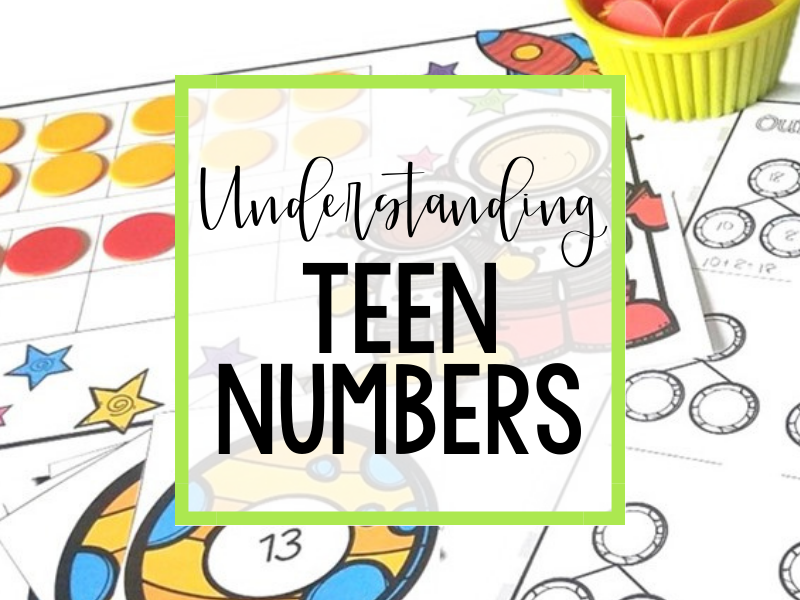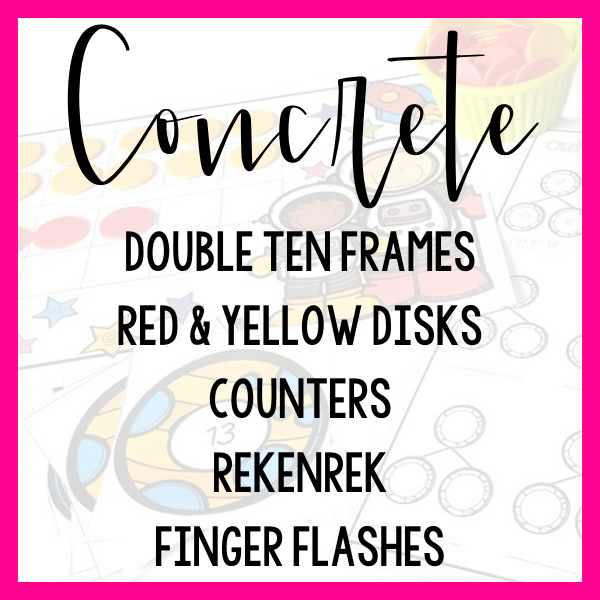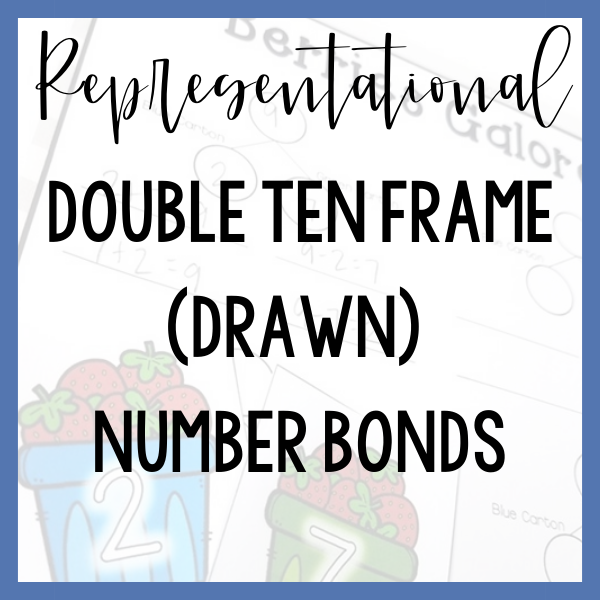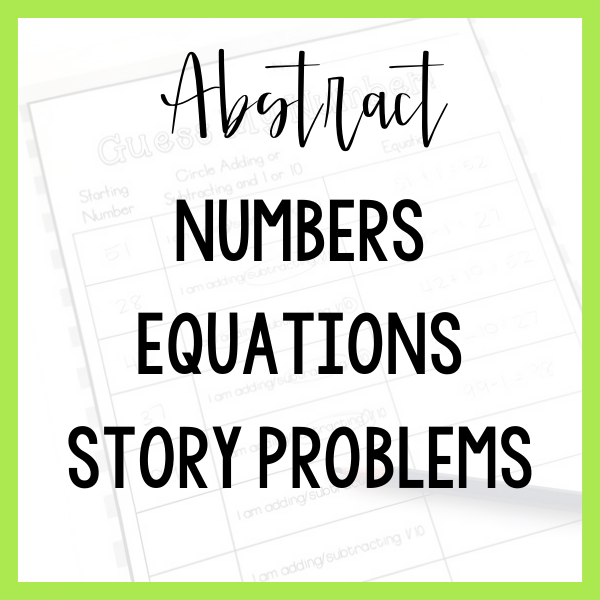# Exploring Teen Numbers

First graders are meant to build upon their understanding of numbers and to build an understanding of place value. It is assumed that first grade students have a strong understanding of teen numbers but that is not always the case! Helping your students to understand that teen numbers are composed of a ten and some ones can be a strong first step in building number and place value knowledge!The 5 examples listed are certainly not an exhaustive list of tools and lessons that could be used to teach this skill.

They are rather a sample progression from hands-on to abstract thinking!

Which step represents your students’ current level of understanding?

As you start your exploration, don’t expect students to see 10 ones as a “ten” right away! Expect that students will start by recognizing these 10 counters as 10 individual items. You can build this understanding through repeated exposure and activities that highlight that teen numbers have a ten and some ones.

Begin by giving each student a double ten frame and a pile of counters. Ask your students to build the number 17 on the double ten frame. This will likely take them some time and that is completely fine. We are building an understanding and there’s no need to rush through this step.

After each of your students have built the number 17 ask them what they notice about what they have built. If your students don’t recognize that there is a full ten and some more ones, simple questions such as “How many counters are up here in the first ten frame?” “How many counters are in this other ten frame?” “What do you notice about the numbers?”

Repeat this activity MANY times. Your students will start to notice that they can build each new number without replacing any counters on the first ten frame. Your students are starting to internalize that teen numbers are made of a ten and some ones.

Connect this activity to a representational model by recording the numbers built in the ten frame on a number bond showing a ten part, a ones part and the whole teen number in the whole.

You can continue to use a double ten frame with your students while reinforcing that a teen number is composed of a ten and some ones. Pre-fill the first ten frame with 10 items in picture form so that they are not movable. Your students are then tasked with adding ones to create each teen number!

This simple adjustment to the activity will further solidify to your students that each teen number has “a ten” and some ones.

As your students are building a variety of numbers on the pre-grouped double ten frame you can still count each of the counters individually to “check their work” and reinforce that, for example, a ten and 3 ones is the same as 13 ones.

Show your students as you slide across all 10 beads on the top row and three beads on the bottom row. Without counting the beads, ask your students if they can tell you how many beads you slid across in all.

Write down your students guesses. If your students were all accurate right away, ask if they can explain how they knew that there were 13 beads. If your students gave you a variety of answers, count the beads to confirm the correct answer. Ask the students who were correct to explain how they knew how many beads you slid across.

Give each student a whiteboard. Ask them to draw a number bond and to write an equation that each relate to the 13 beads you examined on the rekenrek.

Asking your students to relate these visual models to a number bond and an equation so that you can help your students to move away from the need for a visual model so that they are fluent in their ability to compose and decompose teen numbers.

Prepare cards with representations of teen numbers such as a double ten frame, pictures of beads on a rekenrek, fingers on a hand or tally marks.

Choose a card and quickly show the image to the students. Show each representation for only around 5 seconds. If you need to adjust this timing based on the students in your group, go ahead and make a judgment call as needed!

After you have flashed the picture ask your students what number they saw represented. Ask your students how they know what number they saw.

Finally, ask each student to show a number bond and equation on a whiteboard related to the picture shown.

This activity is meant to be completed rapid-fire style. This means that from picture flash -> predictions -> explanation -> whiteboard drawing, each example should only take 1-2 minutes maximum.

Present your students with a word problem that lends itself to indicate a set of 10 and some ones. As your students understand and solve the problem, look for and notice students who quickly and automatically are able to solve the story because of their understanding of teen numbers.

For example: Jacob is stacking cans in the pantry. One shelf is completely full with 10 cans. The next shelf has six cans. How many cans are in the pantry all together?

1.Tell students story problem.

2.Ask students who (or what) was in the story and what they were doing.

3.Ask students to draw a picture using circles that matches the story.

4.Ask students to describe how their picture matches the story.

5.Ask students to draw a number bond and to put the numbers from this story in their number bond.

6.Ask students to write a corresponding equation & solve.

# Tools To USe